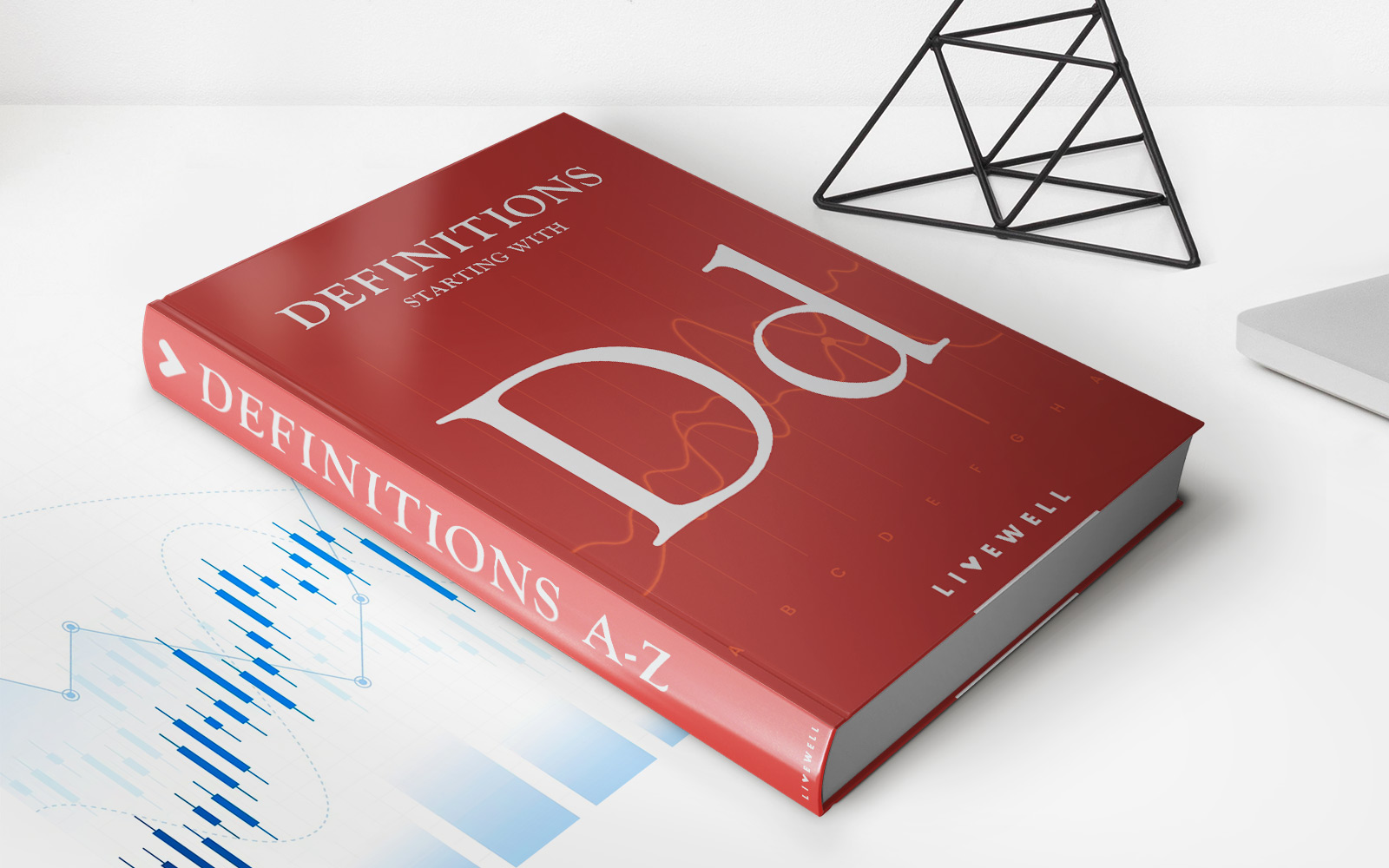Home>Finance>Dividend Payout Ratio Definition, Formula, And CalculationFinance

# Dividend Payout Ratio Definition, Formula, And Calculation

Learn the definition, formula, and calculation of the dividend payout ratio in finance. Understand how this key financial metric can be used to evaluate a company's dividend policy and financial stability.

## Understanding the Dividend Payout Ratio: Definition, Formula, and Calculation

Do you ever wonder how companies distribute their profits to their shareholders? One way companies achieve this is by using the dividend payout ratio. In this blog post, we will explore what the dividend payout ratio is, how it is calculated, and why it is important for investors. If you want to dive deeper into the world of finance, you’re in the right place! Let’s get started!

## Key Takeaways:

• The dividend payout ratio indicates the percentage of a company’s earnings that are paid out as dividends to shareholders.
• This ratio is essential for investors as it helps them assess the sustainability and growth potential of a company’s dividends.

## What is the Dividend Payout Ratio?

The dividend payout ratio is a financial metric that represents the proportion of a company’s earnings that are distributed as dividends to its shareholders. It is expressed as a percentage and reflects how much of the company’s profits are being returned to investors.

## How to Calculate the Dividend Payout Ratio?

The formula to calculate the dividend payout ratio is simple:

Dividend Payout Ratio = Dividends Paid / Earnings

To calculate the dividend payout ratio, you need two pieces of information:

1. Dividends Paid: This refers to the total amount of dividends distributed by the company during a specific period, such as a quarter or a year. This information can typically be found in a company’s financial statements or annual reports.
2. Earnings: Earnings, also known as net income or profit, represent the total profits made by the company during the same period. Again, this information can be found in the company’s financial statements or annual reports.

Once you have these figures, divide the dividends paid by the earnings and multiply the result by 100 to get the dividend payout ratio expressed as a percentage.

## Why is the Dividend Payout Ratio Important?

The dividend payout ratio is a crucial metric for both investors and companies. Here’s why:

1. Assessing Dividend Sustainability: The dividend payout ratio helps investors evaluate whether a company can sustain its current dividend policy. A high ratio may indicate that a company is distributing a large portion of its earnings as dividends, leaving less money for reinvestment or future growth. Conversely, a low ratio may suggest that the company is retaining a significant portion of profits for reinvestment.
2. Evaluating Growth Potential: Companies with a lower dividend payout ratio retain a larger portion of earnings, which can be reinvested in the business. This retained earnings can be used for expanding operations, research and development, or acquisitions, all of which can contribute to future growth. Therefore, analyzing the dividend payout ratio can provide insights into a company’s potential for long-term growth.

By understanding the dividend payout ratio, investors can make informed decisions about their investment portfolio, considering both current income and future growth prospects.

Now, armed with the knowledge of what the dividend payout ratio is, how to calculate it, and why it matters, you are better equipped to analyze potential investment opportunities. Remember, this ratio is just one piece of the puzzle, and it is essential to consider other factors and conduct thorough research before making any investment decisions.

• https://livewell.com/finance/dividend-payout-ratio-definition-formula-and-calculation/